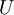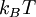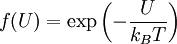Boltzmann factor

The Boltzmann factor of any energy$U$ is defined by the negative exponential of the ratio of the energy to the thermal energy$k_BT$:$f(U)= \exp\left(-\frac{U}{k_BT}\right)$
•$k_B$ is the Boltzmann constant.
•$T$ is the temperature.